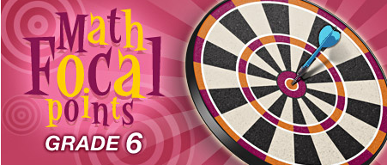### Fractions and Decimals

Students use the meanings of fractions, multiplication and division, and the inverse relationship between multiplication and division to make sense of procedures for multiplying and dividing fractions and explain why they work. They use the relationship between decimals and fractions, as well as the relationship between finite decimals and whole numbers (i.e., a finite decimal multiplied by an appropriate power of 10 is a whole number), to understand and explain the procedures for multiplying and dividing decimals. Students use common procedures to multiply and divide fractions and decimals efficiently and accurately. They multiply and divide fractions and decimals to solve problems, including multistep problems and problems involving measurement (NCTM, 2006, p.18).

These resources offer support in explaining the difficult concepts underpinning multiplication and division of both fractions and decimals. Visual, interactive models are provided where possible. You will also find opportunities for your students to practice their skills in this area of arithmetic.

Multiplication of Fractions Visualize and practice multiplying fractions using an area representation. With the “Show Me” option selected, the virtual manipulative is used to graphically demonstrate, explore, and practice multiplying fractions. A rectangular grid, representing a whole, shows the areas of two fractions to be multiplied, one fraction in red on the left and another in blue at the bottom. The area of the overlapping region, shown in purple, represents the product of their multiplication. The “Test Me” option provides problems to be solved using the same graphical representation.

Multiplying Fractions This tutorial site offers instruction as well as practice in multiplication of fractions. The fractions are modeled with either circles or lines (rectangular areas). The visual display matched with the numerical makes an effective demonstration.

Divide Fractions This tutorial site offers instruction as well as practice in multiplication of fractions. The fractions are modeled with either circles or lines (rectangular areas). The visual display matched with the numerical makes an effective demonstration.

Divide and Conquer This lesson is based on the idea that middle school students can better understand the procedure for dividing fractions if they analyze division through a sequence of problems. Students start with division of whole numbers, followed by division of a whole number by a unit fraction, division of a whole number by a non-unit fraction, and finally division of a fraction by a fraction. Activity sheets and guiding questions are included.

Dividing Fractions Students divide fractions using area models. They can adjust the numerators and denominators of the divisor and dividend and see how the area model and calculation change. Full access to ExploreLearning is available through an annual subscription, but you can apply for a month’s free access in order to test out the applets.

Decimals This site has explanatory lessons and interactive practice on most aspects of decimals, including multiplying decimals and dividing them.

Learning about Multiplication Using Dynamic Sketches of an Area Model In this applet, a rectangle represents the familiar area model of multiplication. By changing the height of the rectangle, students can explore the effect of multiplying a fixed positive number, in this case 3, by decimal numbers greater than 1 and less than 1. The visual is powerful!

Too Big or Too Small? Of these three activities on developing number sense, go to Activity 3: Exploring the Effect of Operations on Decimals. Through playing the cleverly crafted game presented here, students explore the effect of addition, subtraction, multiplication, and division on decimal numbers. Students begin with the number 100 as they enter a maze. For each segment chosen on the maze, the student keys in the assigned operation and number; for example, “+ 1.2” or “? 0.8.” The goal is to choose a path through the maze that results in the largest value at the finish. A copy of the maze playing board is included.

Non-profit Tax ID # 203478467